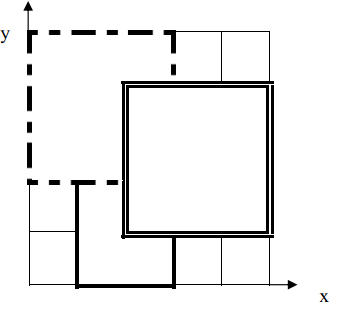GabrielxD

• 累计撰写 673 篇文章
• 累计创建 128 个标签
• 累计收到 15 条评论

### 目 录CONTENT# 【模拟, 枚举】铺地毯2023-04-26 / 0 评论 / 0 点赞 / 152 阅读 / 1,294 字

## 题目

P1003 [NOIP2011 提高组] 铺地毯

$n + 2$ 行包含两个整数 $x$$y$，表示所求的地面的点的坐标 $(x, y)$

3
1 0 2 3
0 2 3 3
2 1 3 3
2 2


3


3
1 0 2 3
0 2 3 3
2 1 3 3
4 5


-1


【样例解释 1】【数据范围】

NOIP2011 提高组 day1 第 $1$ 题。

## 解题

### 方法一：模拟 枚举

#### 代码

import java.util.*;
import java.io.*;

public class Main {
public static void main(String[] args) throws IOException {
in.nextToken(); int n = (int) in.nval;
int[][] carpets = new int[n + 1];
for (int i = 1; i <= n; ++i) {
in.nextToken(); carpets[i] = (int) in.nval;
in.nextToken(); carpets[i] = (int) in.nval;
in.nextToken(); carpets[i] = (int) in.nval + carpets[i];
in.nextToken(); carpets[i] = (int) in.nval + carpets[i];
}
in.nextToken(); int x = (int) in.nval;
in.nextToken(); int y = (int) in.nval;
for (int i = n; i >= 1; --i) {
int x1 = carpets[i], y1 = carpets[i], x2 = carpets[i], y2 = carpets[i];
if (x >= x1 && x <= x2 && y >= y1 && y <= y2) {
System.out.println(i);
return;
}
}
System.out.println(-1);
}
}


import java.util.*;
import java.io.*;

public class Main {
public static void main(String[] args) throws IOException {
in.nextToken(); int n = (int) in.nval;
int[][] carpets = new int[n];
for (int i = 0; i < n; ++i) {
in.nextToken(); carpets[i] = (int) in.nval;
in.nextToken(); carpets[i] = (int) in.nval;
in.nextToken(); carpets[i] = (int) in.nval;
in.nextToken(); carpets[i] = (int) in.nval;
}
in.nextToken(); int x = (int) in.nval;
in.nextToken(); int y = (int) in.nval;
int ans = -1;
for (int i = 0; i < n; ++i) {
int x1 = carpets[i], y1 = carpets[i], x2 = x1 + carpets[i], y2 = y1 + carpets[i];
if (x >= x1 && x <= x2 && y >= y1 && y <= y2) ans = i + 1;
}
System.out.println(ans);
}
}

0